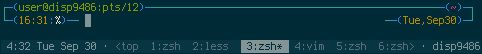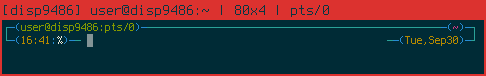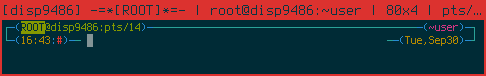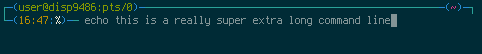In which one geek demonstrates his zsh prompt, which (he hopes) should serve as an example of some of the more complicated aspects of zsh.

A while back, Slashdot had an Ask Slashdot question about shell prompts.  I responded, posting a link to an earlier Kuro5hin post I had made on the subject.  Unlike that Kuro5hin post, the Slashdot post garnered some attention, so I decided to write up how my prompt works.  Since then, I’ve expanded my prompt to take advantage of a lot of zsh stuff, so it should also provide examples to people curious about that sort of thing.For the impatient, I have a screenshot and the prompt command.  For those wanting more detail, I go into it below.

My shell is zsh.  I got the initial idea for this prompt from the “termwide” prompt in the Bash Prompt HOWTO, but had to modify it to work with zsh.  Since then, I’ve added other features (some of which I’ve also seen in other termwide-based prompts) and taken more advantage of zsh’s facilities.  This prompt is now fairly zsh-specific.

Let’s start at the beginning.

function precmd {


There are a number of prompt variables I want to be set before each prompt, so I change them in the special zsh function named precmd.  This is run before the prompt is displayed.

    local TERMWIDTH
(( TERMWIDTH = ${COLUMNS} - 1 ))  local tells zsh that the given variable should be scoped to the current function. I don’t really like cluttering namespaces. The (( ... )) construct tells zsh to carry out mathematical operations. I set the terminal width to one less that the actual width because zsh’s right-hand prompt leaves one character to its right and I want things to line up.  ### # Truncate the path if it's too long. PR_FILLBAR="" PR_PWDLEN="" local promptsize=${#${(%):---(%n@%m:%l)---()--}} local pwdsize=${#${(%):-%~}} if [[ "$promptsize + $pwdsize" -gt$TERMWIDTH ]]; then
((PR_PWDLEN=$TERMWIDTH -$promptsize))
else
PR_FILLBAR="\${(l.(($TERMWIDTH - ($promptsize +$pwdsize)))..${PR_HBAR}.)}" fi  This little section is the one that does the width adjusting. The variables that determine string length are … interesting. Working from the inside out: • ${[varname]:-[string]} returns the value of ${[varname]} normally, but uses [string] instead if${[varname]} doesn’t exist.  ${:-[string]} is a quick way to do variable-related things to fixed strings. • ${([flags])[varname]} uses the flags to alter how the value of the variable is handled.  The percent sign causes prompt expansion to be done on the variable.
• So ${(%):-%~} does prompt expansion on the literal string “%~”. (To get just this effect, print -p "%~" would work, too.) • ${#[varname]} gives the length of the value of the variable.  zsh appears to handle the pound sign before applying the (%) flag, so I had to nest the (%) flag in order to get things to happen in the right order.

If adding the current directory would make the prompt wider than the terminal, I just set $PR_PWDLEN to the length it should be and let zsh truncate it. The actual prompt contains the string %$PW_PWDLEN<...<%~%<<.  When zsh encounters a prompt escape of the form %[num]<[str]<, it will truncate the next part of the prompt until the next %<< or the end of the prompt, whichever comes first.  If truncation is necessary, zsh will remove the beginning of the string to be truncated and add the [str] supplied so that the entire resulting string will be [num] characters long.

(Note that there’s also the %[num]>[str]> sequence, which does the truncation at the end of the string.)

If the combination of invariant prompt and current directory is not wide enough to fill the terminal (this is usually the case), I use another variable flag—one a bit more complicated.  The general form of the left-hand padding flag is ${(l.[len]..[pad1]..[pad2].)[varname]}. This pads or truncates${[varname]} so that the result is exactly [len] characters long.  If padding is needed, the shell will use [pad2] once, then as many iterations of [pad1] as are needed.  If you don’t need [pad2], it can be omitted, as can [varname].  I omit both, so the effect is that [pad1] is repeated to a length of [len].  The quoting is done so I can put variables inside the flag statement.

    ###
# Get APM info.

if which ibam > /dev/null; then
PR_APM_RESULT=ibam --percentbattery
elif which apm > /dev/null; then
PR_APM_RESULT=apm
fi


If apm or ibam is present, I’ll need its output.  I could do this in the prompt itself, but calling programs from within the prompt clobbers the value of $? (return code of the last command run), so I call all external programs in precmd(), where they can’t do any damage. }  And that’s the end of my precmd() function. Next is preexec(), which is only somewhat related to my prompt, but it’s here anyway. preexec() is run after you press enter on your command but before the command is run. setopt extended_glob preexec () { if [[ "$TERM" == "screen" ]]; then
local CMD=${1[(wr)^(*=*|sudo|-*)]} echo -ne "\ek$CMD\e\\"
fi
}


I use screen for a lot of things.  My preexec() sets the screen window title, if I’m running in a screen.  I have fun with variable expansion to get what I want in the title, which is the name of the program I’m currently running:

Subscripts for arrays can have flags that affect their behavior, just like variables can.  The ‘(w)’ flag causes a regular variable to be treated as an array, with each element of the array being a whitespace-separated word of the variable’s value.  The ‘(r)’ flag changes the way the index works.  It returns the first element of the array that matches the pattern supplied as the index.  In the pattern (which uses extended globbing), ‘^’ negates it, so I get the first element that doesn’t match.  It skips variable assignment, ‘sudo’, and program options.

The -e option to echo isn’t strictly necessary in zsh, but I use it out of habit.I’ve attached to a running screen session, which has a window list in the caption.  You can see that the current window, 3, is at a prompt, window 4 is running vim, and 2 is showing something with less.
setprompt () {


The stuff that only needs to be set once is set in a separate function, which I’ve decided to call setprompt().

    ###
# Need this so the prompt will work.

setopt prompt_subst


prompt_subst is not set by default.  It allows variable substitution to take place in the prompt, so I can just change the contents of certain variables without recreating the prompt every time.

    ###
# See if we can use colors.

if [[ "$terminfo[colors]" -ge 8 ]]; then colors fi for color in RED GREEN YELLOW BLUE MAGENTA CYAN WHITE; do eval PR_$color='%{$terminfo[bold]$fg[${(L)color}]%}' eval PR_LIGHT_$color='%{$fg[${(L)color}]%}'
(( count = $count + 1 )) done PR_NO_COLOUR="%{$terminfo[sgr0]%}"


This section determines whether or not to use color in the prompt.  I use terminfo codes to be as portable as possible across different terminal types.  And the zsh termcap module provides an associative array for all of the terminfo entries for the current terminal. ‘sgr0’ removes all attributes (bold, underline, etc.) from the text.  ‘bold’ turns on bold text.  ‘colors’ lists the number of colors the current terminal supports.

The colors module provides a function called colors, which creates associative arrays $fg and$bg, which contain the terminal-appropriate ANSI escape codes for setting the forground and background colors, respectively.  Since the arrays are indexed by the lowercase versions of the color names, I use the (L) flag in the parameter expansion for $color to lower-case the value of that variable. I’ve noticed that colors seems to always populate the arrays regardless of the color support of the terminal, which is why I have the test for the number of supported colors. I fear it may only do ANSI colors as well, but I have yet to use zsh on a terminal that didn’t use ANSI escapes for setting the colors. The escape codes are surrounded by %{ and %}. These are zsh prompt escapes that tell the shell to disregard the contained characters when determining the length of the prompt. This allows zsh to properly position the cursor.Prompt with colors removed.  ### # See if we can use extended characters to look nicer. typeset -A altchar set -A altchar${(s..)terminfo[acsc]}
PR_SET_CHARSET="%{$terminfo[enacs]%}" PR_SHIFT_IN="%{$terminfo[smacs]%}"
PR_SHIFT_OUT="%{$terminfo[rmacs]%}" PR_HBAR=${altchar[q]:--}
PR_ULCORNER=${altchar[l]:--} PR_LLCORNER=${altchar[m]:--}
PR_LRCORNER=${altchar[j]:--} PR_URCORNER=${altchar[k]:--}


Some terminals use fonts that have extended character support.  If they do, there should be terminfo entries to: a) enable use of the line-drawing character set (enacs), b) enter (smacs) and leave (rmacs) the alternate character set, and c) describe the mappings of line drawing characters (acsc).  The last needs some additional explanation.  The VT100 used the alternate character set with certain lowercase characters to make line-drawing characters.  For instance, “q” was a horizontal line.  The acsc terminfo string is a series of character pairs, with the first in the pair being the vt100 character and the second being the character to get the same result in the current terminal.

A zsh associative array is a natural way to get at the appropriate line drawing characters.  Associative arrays must be declared before use, so that’s what the typeset -A does.  (-A is for defining an associative array.)  set -A [arrayname] assigns values to the array, with keys and value alternating.  (key, value, key, value, etc.)  This is exactly how the entries in terminfo are arranged, but we need spaces between the entries.  The (s.[pattern].) flag causes a variable to be split on every occurence of [pattern].  In my case, there is no pattern, so it matches everywhere, splitting between every character.

${[varname]:-[string]} returns, this time with an actual [varname]. So if the terminal doesn’t support line drawing characters, the prompt will fall back to simple dashes.Prompt without line art.  ### # Decide if we need to set titlebar text. case$TERM in
xterm*)
PR_TITLEBAR=$'%{\e]0;%(!.-=*[ROOT]*=- | .)%n@%m:%~ |${COLUMNS}x${LINES} | %y\a%}' ;; screen) PR_TITLEBAR=$'%{\e_screen \005 (\005t) | %(!.-=[ROOT]=- | .)%n@%m:%~ | ${COLUMNS}x${LINES} | %y\e\\%}'
;;
*)
PR_TITLEBAR=''
;;
esac


There are several things going on here. Most generally, I’m setting the titlebar text in terminals that support it.  xterm and xterm-alike terminal emulators support a particular escape sequence to set their titlebar contents.  screen uses a different escape sequence to set its hardstatus line.  (And I have my .screenrc set up to display the hardstatus in xterm’s title bar—details to be linked.)

I’m also using a special zsh prompt escape.  %([char].[true str].[false str]) is a conditional expression.  If [chr] is an exclamation point, zsh will use [true str] if the current uid is that of root and [false str] otherwise.  Since I want to be root as little as possible, I want zsh to yell at me a lot to remind me if I am.

Finally, the whole string is inside $'...' delimiters. This causes the string to be parsed like echo -e would do it (so I can put in “\e” instead of literal escape characters). No other expansion is done, which is also what I want—$COLUMNS and $LINES should only be processed when the prompt is displayed, to deal with changing window sizes.Note the title bar. The “[disp8443]” is added by my window manager, but the rest is from zsh.Here’s the same thing, but inside a screen. Here, the additional screen information is also present in the titlebar. (There’s also my screen caption at the bottom, but that’s unrelated to my shell prompt.)And finally, here’s the titlebar if I’m root. (Don’t worry about the other changes; I’ll get to those.)  ### # Decide whether to set a screen title if [[ "$TERM" == "screen" ]]; then
PR_STITLE=$'%{\ekzsh\e\\%}' else PR_STITLE='' fi  This is the compliment to my preexec() function. It sets the screen title to “zsh” when sitting at a command prompt.  ### # APM detection if which ibam > /dev/null; then PR_APM='$PR_RED${${PR_APM_RESULT[(f)1]}[(w)-2]}%%(${${PR_APM_RESULT[(f)3]}[(w)-1]})$PR_LIGHT_BLUE:' elif which apm > /dev/null; then PR_APM='$PR_RED${PR_APM_RESULT[(w)5,(w)6]/\% /%%}$PR_LIGHT_BLUE:'
else
PR_APM=''
fi


This is for laptops or other computers with batteries.  I assume that if apm or ibam is installed, it’s meant to be used.  These will result in the battery percentage and time left (either to charge or discharge) being displayed in the prompt.

With ibam, that information is on two separate lines.  The percentage is on the first line, so I use ${PR_APM_RESULT[(f)1]} to get just that line. The (f) flag causes the variable to be treated as an array, with each line being a separate element. Then ${...[(w)-2]} returns the second-to-last word on that line.  Similar indices retrieve the last word on the third line.

apm is a bit simpler.  Everything is on a single line, so we just grab the fifth and sixth words on that line.  When two indices are separated by a comma, the result is the range of elements between those two, inclusive.  This just happens to be a two element range.  Then substitution is performed to remove the space between them and to double the percent sign, so that prompt expansion witll leave a single, literal percent sign.  ${[varname]/[pattern]/[replacement]} replaces the first occurrence of [pattern] with [replacement]. ${[varname]/[pattern]//[replacement]} replaces every occurrence.Prompt on my laptop, showing 50% battery and just over two hours to finish recharging.  (No, no indication of AC status; that’s planned.  (I usually know which it is.))
    ###
# Finally, the prompt.

PROMPT='$PR_SET_CHARSET$PR_STITLE${(e)PR_TITLEBAR}\$PR_CYAN$PR_SHIFT_IN$PR_ULCORNER$PR_BLUE$PR_HBAR$PR_SHIFT_OUT(\$PR_GREEN%(!.%SROOT%s.%n)$PR_GREEN@%m:%l\$PR_BLUE)$PR_SHIFT_IN$PR_HBAR$PR_CYAN$PR_HBAR${(e)PR_FILLBAR}$PR_BLUE$PR_HBAR$PR_SHIFT_OUT(\
$PR_MAGENTA%$PR_PWDLEN<...<%~%<<\
$PR_BLUE)$PR_SHIFT_IN$PR_HBAR$PR_CYAN$PR_URCORNER$PR_SHIFT_OUT\
$PR_CYAN$PR_SHIFT_IN$PR_LLCORNER$PR_BLUE$PR_HBAR$PR_SHIFT_OUT(\
%(?..$PR_LIGHT_RED%?$PR_BLUE:)\
${(e)PR_APM}$PR_YELLOW%D{%H:%M}\
$PR_LIGHT_BLUE:%(!.$PR_RED.$PR_WHITE)%#$PR_BLUE)$PR_SHIFT_IN$PR_HBAR$PR_SHIFT_OUT\$PR_CYAN$PR_SHIFT_IN$PR_HBAR$PR_SHIFT_OUT\$PR_NO_COLOUR '


Here’s where everything is finally assembled.  There are a few things in the prompt itself that I haven’t yet explained completely:

I’m using the (e) flag on many variables to cause them to undergo variable substitution.  (That way, things like $COLUMNS and$LINES are updated automatically.)

The %([char].[true str].[false str]) setup returns in several places.  root gets a slightly more obvious prompt that a normal user, as you can see in the previous screenshot.  Also, using a question mark as the [char] allows me to display the exit code of the previous command.  (The true string would be displayed if the exit code was zero, but I have left that string blank.)I ran perl -e 'exit 42'.  It dutifully returned 42, which was then shown in the prompt.

The %D{...} construct results in a date string formatted by the contents.  The current hour and minute go on the left side.

    RPROMPT=' $PR_CYAN$PR_SHIFT_IN$PR_HBAR$PR_BLUE$PR_HBAR$PR_SHIFT_OUT\
($PR_YELLOW%D{%a,%b%d}$PR_BLUE)$PR_SHIFT_IN$PR_HBAR$PR_CYAN$PR_LRCORNER$PR_SHIFT_OUT$PR_NO_COLOUR'


zsh supports a right-hand prompt, too.  I started using it just because it was there, but I’ve come to like it.  Putting stuff on the right frees up space on the left, and the right-hand prompt simply disappears if the command line grows past it.  Thus, I put information that would be useful but that I don’t need all that often on the right.The right-hand prompt has been removed to make room for the command line.
    PS2='$PR_CYAN$PR_SHIFT_IN$PR_HBAR$PR_SHIFT_OUT\
$PR_BLUE$PR_SHIFT_IN$PR_HBAR$PR_SHIFT_OUT(\
$PR_LIGHT_GREEN%_$PR_BLUE)$PR_SHIFT_IN$PR_HBAR$PR_SHIFT_OUT\$PR_CYAN$PR_SHIFT_IN$PR_HBAR$PR_SHIFT_OUT$PR_NO_COLOUR '


PS2 is the continuation prompt.  I define it mostly so it will match the main one in color.  In it, I use the %_ prompt escape, which shows the reason the continuation prompt is being displayed.

}